# 11 Different Types Of Forces

In physics, a force can be defined as a push or pull on any object that has mass. It changes the object’s motion.

In other words, a force causes an object with mass to change its direction and velocity.

Two great physicists Isaac Newton and Galileo Galilei described the behavior of forces mathematically. In 1638, Galileo conducted an incline-plane experiment that revolutionized the way of measuring force. Five decades later, Newton came up with the Laws of Motion, which laid the foundation for classical mechanics.

Since force has both magnitude and direction, it is a vector quantity. It is represented by the symbol F and measured in the SI unit of Newton (N).

Forces can be categorized into two groups based on their applications:

1. Contact Force: acts on a body either directly or through a medium.
2. Non-Contact Force: acts through spaces without making direct contact with the body.

To better explain the phenomenon, we have described all different types of forces with examples. Let’s start with the four fundamental forces in nature.

### 1. Gravitational Force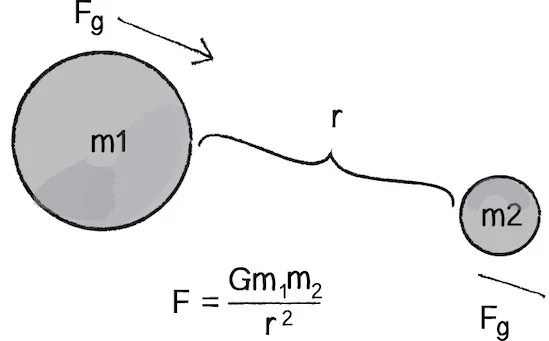G is the universal gravitational constant, which varies with different astronomical bodies

Type: Non-contact force

Gravitational force is something that attracts two objects with mass. It acts on every object, including you, in the universe.

The magnitude of the gravitational force objects exert on each other is “directly proportional to the product of their masses and inversely proportional to the square of the distance between them”. The more massive the objects and the lesser the distance between them, the higher the force.

It is the weakest of the four fundamental forces found in nature.

While the gravitational force has no substantial influence at subatomic scales, it is the dominant interaction at the macroscopic scale, and it greatly affects the formation, structure, and trajectory of celestial bodies.

Example: Gravity causes an apple to fall down from a tree; it causes the moon to revolve around the Earth; it holds the gases in the Sun.

### 2. Electromagnetic Force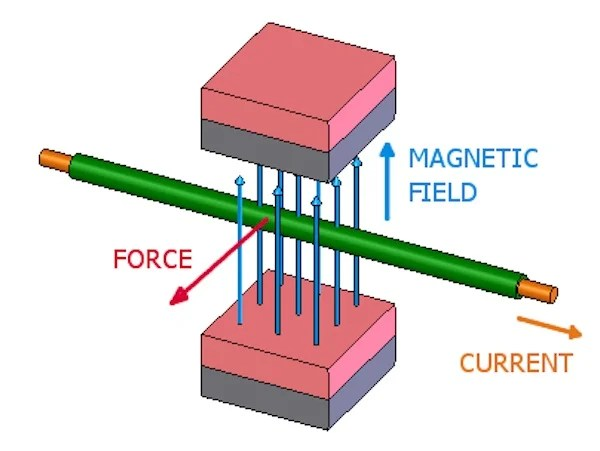Type: Non-contact force

It is a type of interaction that occurs between electrically charged particles. Electromagnetic fields (produced by moving electric charges) carry the electromagnetic force.

Electricity and magnetism are associated with each other: flowing electrons create magnetism and moving magnets generate electricity. The relationship between the two is very well explained by James Clerk Maxwell and is quantified in his equations.

Example: The most common example of electromagnetism is light, as it propagates (radiates) through space, carrying electromagnetic radiant energy.

The next most common example would be the forces acting between the electrically charged atomic nuclei and electrons of the atoms.

### 3. Strong Nuclear Force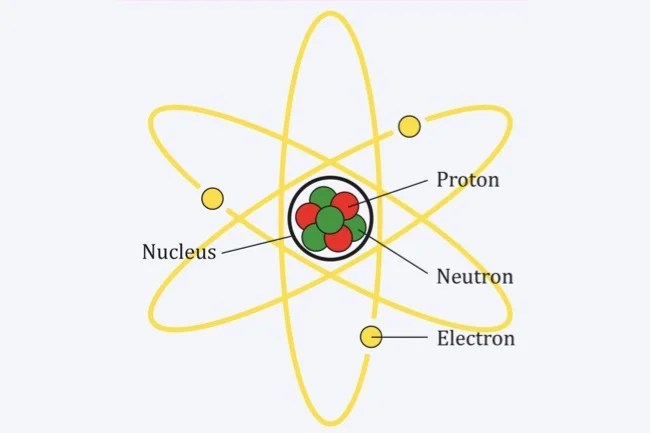Protons and neutrons held by the strong nuclear force

Type: Non-contact force

In nuclear and particle physics, the strong force is responsible for the structural integrity of atomic nuclei. Since all protons have a positive charge, they repel each other. The strong nuclear force holds these repelling protons together so that they can form an atomic nucleus.

About 99% of the mass of a neutron or proton is the result of the strong force field energy.

It is the strongest force in nature that acts at the range of 1 femtometer (10−15 m). It is nearly 137 stronger than electromagnetism and 100 undecillion (1038) times stronger than the gravitational force.

Example: The strong nuclear force binds quarks into hadron particles, such as the proton and neutron, to create an atomic nucleus. This the force that holes ordinary matter together.

On a larger scale, it is used in nuclear power plants to produce heat for the purpose of generating electricity. It is also responsible for the massive destructive power of nuclear weapons. Because of this force, nuclear weapons, when detonated, release an extreme amount of energy.

### 4. Weak Nuclear Force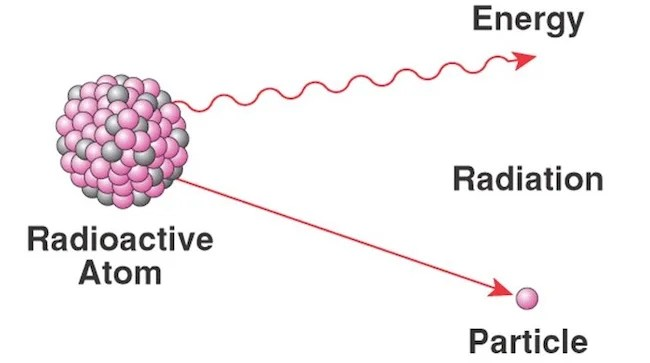Radioactive decay of a particle

Type: Non-contact force

In nuclear physics, the weak force refers to the interaction between subatomic particles that causes radioactive decay of atoms. More specifically, it is responsible for the decay of some nucleons into leptons and other types of hadrons.

Its field strength is approximately 1013 times less than that of the strong nuclear force. However, it is significantly stronger than the gravitational force over short distances.

Example: The most familiar effect of the weak force is beta decay (of neutrons) and the associated radioactivity. It occurs in several different reactions, including the burning of the Sun and radiocarbon dating.

These are the four fundamental (non-contact) forces from which everything else is derived. They keep the stars burning and planets spinning. Without them, the universe we know wouldn’t exist, and even if it did, it would be a completely different place.

Now let’s move on to non-fundamental forces that result from direct physical interaction between two objects.

### 5. Applied ForceType: Contact force

As the name suggests, it is the force that you apply to an object. The object begins to move when the magnitude of the force overcomes the inertia of the object.

The body remains at rest or in uniform motion in a straight line unless an external force is applied to them, which changes the body’s state of motion and direction. The acceleration of the body is directly proportional to the applied force.

Example: The force exerted on a box by a person.

### 6. Frictional ForceType: Contact force

The surface force that opposes the relative motion of a body is called frictional force. Since no object is perfectly smooth in the real world, there is always some friction between two surfaces. Its magnitude is proportional to the coefficient of friction of the surface’s material.

The two major types of frictional forces are static (force of friction on a resting object) and kinetic friction (force of friction on a moving object). Air resistance is also a frictional force that acts upon objects as they travel through the air.

It always acts in the opposite direction to the motion and converts kinetic energy into thermal energy (work to heat). All in all, friction is a crucial and desirable force that supplies traction to facilitate motion on land.

Example: A coaster sliding against a table, two cards in a deck sliding against each other, and rubbing your hand to generate heat, are all examples of friction.

### 7. Normal Force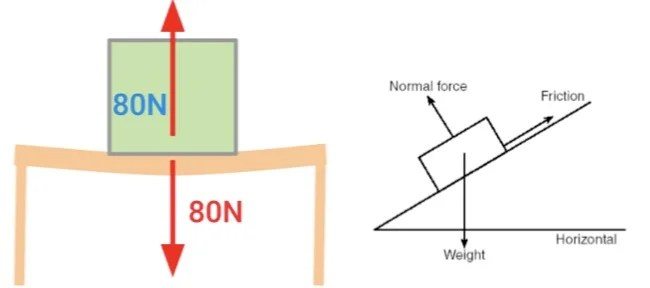Type: Contact force

When two surfaces are in contact, they exert a normal force on each other. The term ‘normal’ refers to perpendicular. This means the force is directed perpendicular to the two surfaces in contact.

Example: When a laptop is placed on a table, the normal force keeps it from falling through the table. The Earth’s gravitational force pulls the laptop downward, but since it doesn’t actually fall, there must be a force constantly pushing it up. That is what we call the normal force.

It comes from the electromagnetic force: the electrons in the laptop push against the table’s electrons. Since all electrons are negatively charged, they do not get much closer to each other, and the laptop rests on the top of the table.

### 8. TensionImage credit: Khan Academy

Type: Contact force

The tension force is usually transmitted through a wire, cable, string, or rope when it is tightly pulled by forces acting from opposite ends. The force is directed along the length of the cable.

Tension can also be defined as the action-reaction pair of forces acting at each end of the cable. It is the opposite of compression.

Example: A rope pulling a box or a box hanging on a rope would be a great example of tension (in the rope).

### 9. Spring ForceType: Contact force

The spring force is the force exerted by a stretched or compressed string upon an object that is attached to it.

The ability of the spring to resist a distorting influence and to return to its original state when influence is removed depends on its material, the number of coils, and the diameter of the wire that forms the coils. Generally, these characteristics are quantified in a parameter called spring constant ‘k’.

For all springs that obey Hooke’s Law, the magnitude of the force is directly proportional to the spring constant (k) and compressed/stretched length (x).

Example: Automobile shock absorbers are made of springs. They are designed to absorb shock impulses by converting the kinetic energy of the shock into another form of energy (such as heat), which is then dissipated.

### 10. Centripetal Force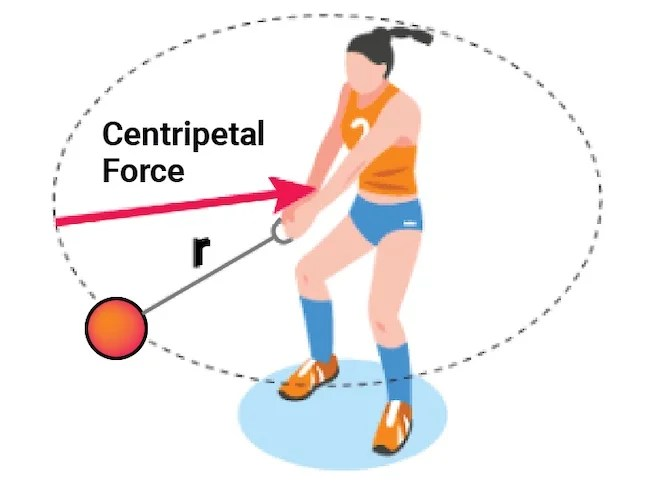Type: Non-contact force

The centripetal force acts on objects accelerating in a circular motion. It is the force that makes an object follow a curved path.

The direction of the force is always towards the fixed point of the instantaneous center of curvature of the path and orthogonal to the object’s motion.

Example: The two most common examples of centripetal force are turning a car and the Earth orbiting around the Sun. In the former case, the centripetal force is provided by the friction between the wheels and the ground, whereas in the latter case, it is provided by the force of gravity.

### 11. Fictitious forces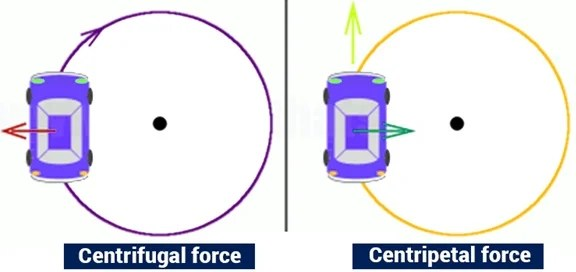Type: Non-contact force

Fictitious forces (also called pseudo forces) are apparent forces that act on masses whose motion is described using a non-inertial frame of reference, including the rotating reference frame.

It comes in effect when the frame of reference has started acceleration. The term ‘pseudo force’ has a precise meaning with Newtonian mechanics – in fact, it is always proportional to the object’s mass on which it acts.

Example: The forces you experience in a moving vehicle are everyday examples of fictitious forces. When the vehicle accelerates in the forward direction, it pushes you back into your seat. When the vehicle makes sharp turns, it throws you side to side. These influences arise due to the fact that the natural frame of reference for a given situation is itself accelerating.

In the rotating reference frame, the fictitious force is the force that appears to push objects outwards towards the rim of a centrifuge.Written by
###### Varun Kumar

Varun Kumar is a professional science and technology journalist and a big fan of AI, machines, and space exploration. He received a Master's degree in computer science from GGSIPU University. To find out about his latest projects, feel free to directly email him at [email protected]